Ask your WordPress questions! Pay money and get answers fast!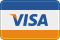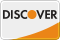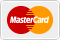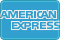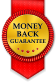# [Shortcode] Get comment count from page via page slug WordPress

• SOLVED

I want a shortcode that can retrieve the comment count from a page by its page slug.

Example: [comment-count page="page-slug"]

The following code does it by page ID:

``` function comments_shortcode(\$atts) { extract( shortcode_atts( array( 'id' => '' ), \$atts ) ); \$num = 0; \$post_id = \$id; \$queried_post = get_post(\$post_id); \$cc = \$queried_post->comment_count; if( \$cc == \$num || \$cc > 1 ) : \$cc = \$cc.' Comments'; else : \$cc = \$cc.' Comment'; endif; \$permalink = get_permalink(\$post_id); return '<a href="'. \$permalink . '" class="comments_link">' . \$cc . '</a>'; } add_shortcode('comments', 'comments_shortcode'); ```

2018-12-28

Try this,
```function comments_shortcode(\$atts) { extract(shortcode_atts(array( 'page' => '' ), \$atts)); \$page_details = get_page_by_path( \$page ); \$num = 0; \$post_id = \$page_details->ID; \$queried_post = get_post(\$post_id); \$cc = \$queried_post->comment_count; if (\$cc == \$num || \$cc > 1) : \$cc = \$cc.' Comments'; else : \$cc = \$cc.' Comment'; endif; \$permalink = get_permalink(\$post_id); return '<a href="'. \$permalink . '" class="comments_link">' . \$cc . '</a>'; } add_shortcode('comment_count', 'comments_shortcode');```

You can add shortcode like this
[comment_count page="page-slug"]

``` function comments_count_func(\$atts) { extract(shortcode_atts(array( 'page' => '', ), \$atts)); \$args = [ 'post_name__in' => [\$page], 'fields' => 'ids' ]; \$q = get_posts( \$args ); \$num = 0; \$post_id = \$q; \$queried_post = get_post(\$post_id); \$cc = \$queried_post->comment_count; if( \$cc == \$num || \$cc > 1 ) : \$cc = \$cc.' Comments'; else : \$cc = \$cc.' Comment'; endif; \$permalink = get_permalink(\$post_id); return '<a href="'. \$permalink . '" class="comments_link">' . \$cc . '</a>'; } add_shortcode('comments-count', 'comments_count_func'); ```
And use this as shortcode
`[comments-count page=”page-slug-here”]`

I have tested this and its working for me.

2018-12-28

Hi,

You can get a page as the object by its slug. After that you can get its ID from the object easily.

For example, for a page with 'about' slug you can do the follwoing:

`\$page = get_page_by_path( 'about' );`

Thanks

I need it as a shortcode specifically.

Plesae replace your complete code with the following code:

``` function comments_shortcode(\$atts) { extract( shortcode_atts( array( 'slug' => '' ), \$atts ) ); \$num = 0; \$page = get_page_by_path( \$slug ); \$post_id = \$page->ID; \$queried_post = get_post(\$post_id); \$cc = \$queried_post->comment_count; if( \$cc == \$num || \$cc > 1 ) : \$cc = \$cc.' Comments'; else : \$cc = \$cc.' Comment'; endif; \$permalink = get_permalink(\$post_id); return '<a href="'. \$permalink . '" class="comments_link">' . \$cc . '</a>'; } add_shortcode('comments', 'comments_shortcode'); ```

Then use the following shortcode with any page slug as slug argument. I used about page slug in the example:

Thanks

2018-12-28

``` function comments_shortcode( \$atts ) { extract( shortcode_atts( array( 'slug' => '' ), \$atts ) ); \$queried_post = get_page_by_path( \$slug ); \$cc = \$queried_post->comment_count; \$cc = \$cc > 1 ? \$cc . ' Comments' : \$cc . ' Comment'; \$permalink = get_permalink( \$queried_post->ID ); return '<a href="' . \$permalink . '" class="comments_link">' . \$cc . '</a>'; } add_shortcode( 'comments', 'comments_shortcode' ); ```

I have refactored a bit for clean code. Better formatted code at: https://gist.github.com/fahdi/73e7bcc107b82e5c66a63ddaf6d7463c

2018-12-28

```function comments_count_func(\$atts) { extract(shortcode_atts(array( 'page' => '', ), \$atts)); \$args = [ 'post_name__in' => [\$page], 'fields' => 'ids' ]; \$q = get_posts( \$args ); \$num = 0; \$post_id = \$q; \$queried_post = get_post(\$post_id); \$cc = \$queried_post->comment_count; if( \$cc == \$num || \$cc > 1 ) : \$cc = \$cc.' Comments'; else : \$cc = \$cc.' Comment'; endif; \$permalink = get_permalink(\$post_id); return '<a href="'. \$permalink . '" class="comments_link">' . \$cc . '</a>'; } add_shortcode('comments-count', 'comments_count_func'); ```
`[comments-count page=”page-slug-here”]`